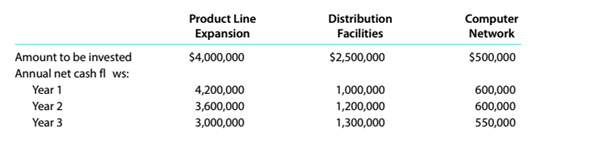Chapter 15, Problem 15.3.3P

Chapter
Section
Textbook Problem

Net present value method, present value index, and analysisDonahue Industries Inc. wishes to evaluate three capital investment projects by using thenet present value method. Relevant data related to the projects are summarized as follows:Instructions Which project offers the largest amount of present value per dollar of investment? Explain.

To determine

Concept Introduction:

NPV:

Net present value (NPV) is the method to evaluate the project feasibility. This method calculates the present value of cash inflows and outflows, and then calculates the net present value of the investment. A project should be accepted if it has a positive NPV. The formula to calculate the NPV is as follows:

NPV = Present value of cash inflows  Present value of cash out flows

To Identify:

The project which is offering the highest amount of present value per dollar of investment

Explanation

The Present value Index for each project is prepared as follows:

 Product Line Expansion Amount PVF (20%) PV A B =A*B Annual Net Cash Flows Year 1 $4,200,000 0.83333$ 3,500,000 Year 2 $3,600,000 0.69444$ 2,500,000 Year 3 $3,000,000 0.57870$ 1,736,111 Present Value of Cash Inflow (A) $7,736,111 Investment (B)$ 4,000,000 1.00000 $4,000,000 Present Value Index (A/B) 1.93 Distribution Facilities Amount PVF (20%) PV A B =A*B Annual Net Cash Flows Year 1$ 1,000,000 0.83333 $833,333 Year 2$ 1,200,000 0...

Still sussing out bartleby?

Check out a sample textbook solution.

See a sample solution

The Solution to Your Study Problems

Bartleby provides explanations to thousands of textbook problems written by our experts, many with advanced degrees!

Get Started

Explain why standard costing systems adopted.

Managerial Accounting: The Cornerstone of Business Decision-Making

When are data in 3NF?

Accounting Information Systems

Operating cash flows rather than accounting income are listed in Table 12.1. Why do we focus on cash flows as o...

Fundamentals of Financial Management, Concise Edition (with Thomson ONE - Business School Edition, 1 term (6 months) Printed Access Card) (MindTap Course List)